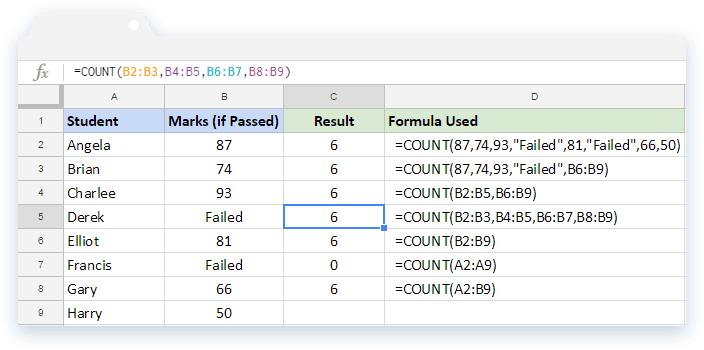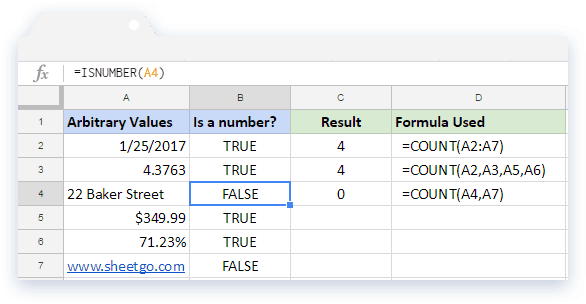# How to use the COUNT formula in Google SheetsThe COUNT formula in Google Sheets is one of the simplest and most widely used formulas for day to day spreadsheet needs. It gives us the number of numeric values within the specified data set. Simply put, it helps us to count numbers.

### Syntax

COUNT(value1, [value2, …])

• value1 – is the value or reference to a range of cell(s) to consider while counting.
• value2 – and above are optional and additional values or ranges to consider for counting.

#### Usage: COUNT formula in Google Sheets

We will try and explain the syntax with a few examples below – as it helps strengthen our understanding.From the list of cases illustrated above, we can deduce that the input parameters can take any of the following forms. It can be a number, text within double quotes, and reference to a range of cells.

Consider the first five cases (rows 2 through to 6). No matter what kind of combination we use for the input parameters, the COUNT formula does its job – just counts the numbers. In all of these cases, the formula returned the value 6, while there are 8 different values (including the “Failed” ones). Therefore, we can establish that it is comfortably ignoring the text values, and only taking numbers into account.

Let us see what other things the COUNT formula counts.In the above image, we have put in various arbitrary values like a date, currency, percentage, decimal, and text. And accordingly we have validated for numbers on the second column, using the ISNUMBER formula. So, the values on the rows 2, 3, 5 and 6 are numbers and those on 4 and 7 are not numbers. And, not surprisingly, the COUNT formulas on the column C counted only those that are numbers.

So far, so good. What if we want to include the non numeric values within the count result? We can do that using another simple function. It is the COUNT formula’s big brother, the COUNTA formula, which is capable of counting text values too. However, we demonstrate this concept in a different post.